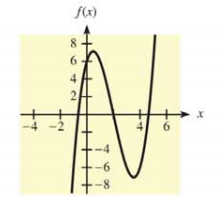Chapter 10.2, Problem 9E### Mathematical Applications for the ...

11th Edition
Ronald J. Harshbarger + 1 other
ISBN: 9781305108042

#### Solutions

Chapter
Section### Mathematical Applications for the ...

11th Edition
Ronald J. Harshbarger + 1 other
ISBN: 9781305108042
Textbook Problem

# In Problems 11-14, a function and its graph are given. Use the second derivative to determine intervals on which the function is concave up, to determine intervals on which it is concave down, and to locate points of inflection. Check these results against the graph shown. f ( x ) = x 3 − 6 x 2 + 5 x + 6To determine

To calculate: The intervals over which the function f(x)=x36x2+5x+6 is concave up, concave down and the points of inflection.

Explanation

Given Information:

The provided function is,

f(x)=x36x2+5x+6

The graph of provided function is,

Formula used:

For a function f(x) whose double derivative is defined, the points of inflection are obtained by the equation,

f(x)=0

Calculation:

Consider the provided function,

f(x)=x36x2+5x+6

Now, obtain the first-order derivative of this function as:

f(x)=x36x2+5x+6f(x)=3x212x+5

Now, obtain the second-order derivative as,

f(x)=3x212x+5f(x)=6x12

Now, set f(x)=0 to obtain points of

### Still sussing out bartleby?

Check out a sample textbook solution.

See a sample solution

#### The Solution to Your Study Problems

Bartleby provides explanations to thousands of textbook problems written by our experts, many with advanced degrees!

Get Started

#### Find more solutions based on key concepts